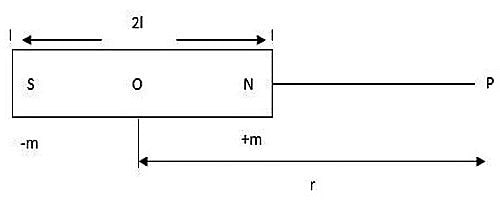# Intensity of Magnetization

The intensity of magnetization represents the extent to which a material has been magnetized under the influence of magnetizing field H.

Definition: The Magnetic instant of a magnet undergoes a transformation when it is positioned in a magnetic field. This alteration that is, the magnetic moment change per unit volume is the Intensity of Magnetization. It represents the extent to which an example is magnetized when placed in a magnetizing field. In other words, the intensity of magnetization of a magnetic substance is defined as the magnetic moment per unit volume of the material.

The intensity of magnetization of a magnetic material is defined as the magnetic moment per unit volume of the material.

I = M/V

Its unit is Am-1.

For a specimen of length 2Ɩ, area A and pole strength m,

I = 2Ɩm / 2ƖA

so, I = m/A

Hence, the intensity of magnetization is also defined as the pole strength per unit area of the cross-section of the material.

General Information on Intensity of Magnetization:

• The intensity of magnetization is denoted by the letter I. Unit of I is Am-1.
• The SI unit of this quantity is the Ampere per meter (A/m).
• It represents the extent to which the substance is magnetized.
• When we place a substance in the magnetic field, atomic dipoles of the material tends to align fully or partially in the direction of the field.
• So the net magnetic moment is developed in the direction of the field in any small volume of the material.
• The intensity of magnetism is defined as the magnetic moment per unit volume of the magnetized material so, I = M/V, where M is the total magnetic moment within volume due to the magnetizing field.Fig: Intensity of Magnetization in Longitudinal Position

The intensity of magnetization represents the extent to which an example is magnetized when placed in a magnetizing field. The net magnetization results from the reaction of a material to the exterior magnetic field, together with any unstable magnetic dipole moment that is inherent in the substance due to the motion in its electrons as mentioned earlier. So, the intensity of magnetization may also be defined as the pole strength developed per unit area of the substances when subjected to a consistent magnetic field. The concept of magnetization helps us in classifying the materials on the foundation of their magnetic property. It indicates how the sample affected by the magnetic field when placed in it. It might be positive or negative depending upon either the magnetization taking place in the direction or in the opposite direction of the magnetizing field.